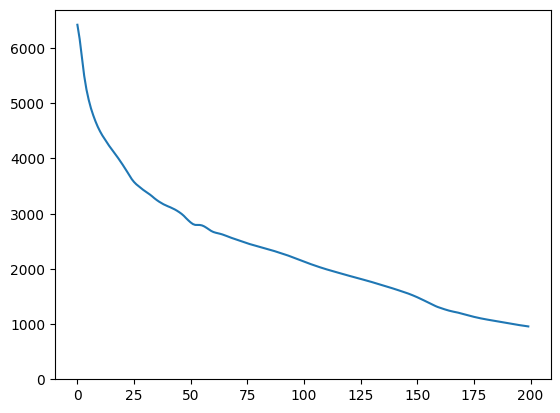# Generate Artificial Faces with CelebA Progressive GAN Model

This Colab demonstrates use of a TF Hub module based on a generative adversarial network (GAN). The module maps from N-dimensional vectors, called latent space, to RGB images.

Two examples are provided:

• Mapping from latent space to images, and
• Given a target image, using gradient descent to find a latent vector that generates an image similar to the target image.

## Optional prerequisites

### More models

Here you can find all models currently hosted on tfhub.dev that can generate images.

## Setup

````# Install imageio for creating animations.`
`pip -q install imageio`
`pip -q install scikit-image`
`pip install git+https://github.com/tensorflow/docs`
```

### Imports and function definitions

```2023-10-09 19:16:36.723734: E tensorflow/compiler/xla/stream_executor/cuda/cuda_dnn.cc:9342] Unable to register cuDNN factory: Attempting to register factory for plugin cuDNN when one has already been registered
2023-10-09 19:16:36.723791: E tensorflow/compiler/xla/stream_executor/cuda/cuda_fft.cc:609] Unable to register cuFFT factory: Attempting to register factory for plugin cuFFT when one has already been registered
2023-10-09 19:16:36.723829: E tensorflow/compiler/xla/stream_executor/cuda/cuda_blas.cc:1518] Unable to register cuBLAS factory: Attempting to register factory for plugin cuBLAS when one has already been registered
```

## Latent space interpolation

### Random vectors

Latent space interpolation between two randomly initialized vectors. We will use a TF Hub module progan-128 that contains a pre-trained Progressive GAN.

``````progan = hub.load("https://tfhub.dev/google/progan-128/1").signatures['default']
``````
```2023-10-09 19:16:40.403155: E tensorflow/compiler/xla/stream_executor/cuda/cuda_driver.cc:268] failed call to cuInit: CUDA_ERROR_NO_DEVICE: no CUDA-capable device is detected
```
``````def interpolate_between_vectors():
v1 = tf.random.normal([latent_dim])
v2 = tf.random.normal([latent_dim])

# Creates a tensor with 25 steps of interpolation between v1 and v2.
vectors = interpolate_hypersphere(v1, v2, 50)

# Uses module to generate images from the latent space.
interpolated_images = progan(vectors)['default']

return interpolated_images

interpolated_images = interpolate_between_vectors()
animate(interpolated_images)
``````## Finding closest vector in latent space

Fix a target image. As an example use an image generated from the module or upload your own.

``````image_from_module_space = True  # @param { isTemplate:true, type:"boolean" }

def get_module_space_image():
vector = tf.random.normal([1, latent_dim])
images = progan(vector)['default']
return images

return transform.resize(image, [128, 128])

if image_from_module_space:
target_image = get_module_space_image()
else:

display_image(target_image)
``````After defining a loss function between the target image and the image generated by a latent space variable, we can use gradient descent to find variable values that minimize the loss.

``````tf.random.set_seed(42)
initial_vector = tf.random.normal([1, latent_dim])
``````
``````display_image(progan(initial_vector)['default'])
````````````def find_closest_latent_vector(initial_vector, num_optimization_steps,
steps_per_image):
images = []
losses = []

vector = tf.Variable(initial_vector)
loss_fn = tf.losses.MeanAbsoluteError(reduction="sum")

for step in range(num_optimization_steps):
if (step % 100)==0:
print()
print('.', end='')
if (step % steps_per_image) == 0:
images.append(image.numpy())
target_image_difference = loss_fn(image, target_image[:,:,:3])
# The latent vectors were sampled from a normal distribution. We can get
# more realistic images if we regularize the length of the latent vector to
# the average length of vector from this distribution.
regularizer = tf.abs(tf.norm(vector) - np.sqrt(latent_dim))

loss = target_image_difference + regularizer
losses.append(loss.numpy())

return images, losses

num_optimization_steps=200
steps_per_image=5
images, loss = find_closest_latent_vector(initial_vector, num_optimization_steps, steps_per_image)
``````
```....................................................................................................
....................................................................................................
```
``````plt.plot(loss)
plt.ylim([0,max(plt.ylim())])
``````
```(0.0, 6696.3041717529295)
`````````animate(np.stack(images))
``````Compare the result to the target:

``````display_image(np.concatenate([images[-1], target_image], axis=1))
``````### Playing with the above example

If image is from the module space, the descent is quick and converges to a reasonable sample. Try out descending to an image that is not from the module space. The descent will only converge if the image is reasonably close to the space of training images.

How to make it descend faster and to a more realistic image? One can try:

• using different loss on the image difference, e.g. quadratic,
• using different regularizer on the latent vector,
• initializing from a random vector in multiple runs,
• etc.
[{ "type": "thumb-down", "id": "missingTheInformationINeed", "label":"Missing the information I need" },{ "type": "thumb-down", "id": "tooComplicatedTooManySteps", "label":"Too complicated / too many steps" },{ "type": "thumb-down", "id": "outOfDate", "label":"Out of date" },{ "type": "thumb-down", "id": "samplesCodeIssue", "label":"Samples / code issue" },{ "type": "thumb-down", "id": "otherDown", "label":"Other" }]
[{ "type": "thumb-up", "id": "easyToUnderstand", "label":"Easy to understand" },{ "type": "thumb-up", "id": "solvedMyProblem", "label":"Solved my problem" },{ "type": "thumb-up", "id": "otherUp", "label":"Other" }]